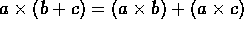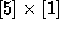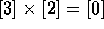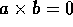Next: Fields Up: Algebraic structures Previous: Monoids and Groups

## Rings

If we take an Abelian group (remember: a set with a binary operation) and we define a second operation on it we get a bit more of a structure than we had with just a group.

If the second operation is associative, and it is distributive over the first then we have a ring. Note that the second operation may not have an identity element, nor do we need to find an inverse for every element with respect to this second operation. As for what distributive means, intuitively it is what we do in math when perform the following change:.

If the second operation is also commutative then we have what is called a commutative ring. The set of integers (with addition and multiplication) is a commutative ring (with even an identity - called unit element - for multiplication).

Now let us go back to our set of remainders. What happens if we multiply? We see that we get , in fact we can see a number of things according to our definitions above,  is its own inverse, and  is the multiplicative element. We can also show easily enough (by creating a complete multiplication table) that it is commutative. But notice that if we take  and , neither of which are equal to the class that the zero belongs to , and we multiply them, we get. This bring us to the next definition. In a commutative ring, let us take an element which is not equal to zero and call it a. If we can find a non-zero element, say b that combined with a equals zero () then a is called a zero divisor.

A commutative ring is called an integral domain if it has no zero divisors. Well the set Z with addition and multiplication fullfills all the necessary requirements, and so it is an integral domain. Notice that our set of remainders is not an integral domain, but we can build a similar set with remainders of division by five, for example, and voilà, we have an integral domain.

Let us take, for example, the set Q of rational numbers with addition and multiplication - I'll leave out the proof that it is a ring, but I think you should be able to verify it easily enough with the above definitions. But to give you a head start, notice the addition of rationals follow all the requirements for an abelian group. If we remove the zero we will have another abelian group, and that implies that we have something more than a ring, in fact, as we will see in the next section.Next: Fields Up: Algebraic structures Previous: Monoids and Groups

Alex Lopez-Ortiz
Mon Feb 23 16:26:48 EST 1998